Robust Modeling of Policy Changes: Difference in Difference (DiD)

The difference in Difference (DiD) is a popular method in empirical economics and has important applications in other social sciences as well. DID is a quasi-experimental design that uses panel data to estimate the effects of specific intervention or treatment (such as policy changes, new laws, social program implementation) on outcomes over time and between two population groups, those that are affected by this policy and those that are not. DiD, in general, is an appealing choice for researchers who want to design a research methodology based on controlling for confounding variables.

Applications of DiD are quite diverse, amongst are

• Impact evaluation (public policy analysis)
• Measuring the variations overtime (Time Series) and over individuals (Cross-Sectional Data)
• Focusing on the establishment of effects on a dependent variable derived from the interaction of exogenous variables given a treatment.
• Variants of the DiD method can account to deal with auto-selection bias and endogeneity problems.
• Comparing the differences between observed outcomes from partial and non-randomized samples in groups.

Although this method is highly important, few learning resources are available to instruct researchers and scientists how to properly implement and design it. There may be some resources that discuss the theoretical foundations of this method while listing a few examples of its applications. However a fully-fledged learning material for DiD that covers both theory, and guides researchers to implement this method on statistical software using real and simulated data applications are rather scarce.

At M&S Research Hub we recently launched a video library wherein our team of academic experts record offline training videos for advanced econometrics methods. This material is designed to fit researchers at different proficiency levels. They cover both theoretical and mathematical basics of the target models and their detailed application using statistical software, leaving the researcher in no further need to search for other learning resources.

A complete DiD course that takes around 158 minutes and is recorded over 7 videos are available in the library for everyone who wants to master the DID method.

Taking Logarithms of Growth Rates and Log-based Data.

A usual practice while we’re handling economic data, is the use of logarithms, the main idea behind using them is to reduce the Heteroscedasticity -HT- of the data (Nau, 2019). Thus reducing HT, implies reducing the variance of the data. Several times, different authors implement some kind of double logarithm transformation, which is defined as taking logarithms of the data which is already in logarithms and growth rates (via differencing logarithms).

The objective of this article is to present the implications of this procedures, first by analyzing what does do the logarithm to a variable, then observing what possible inferences can be done when logarithms are applied to growth rates.

There are a series of properties about the logarithms that should be considered first, we’re not reviewing them here, however the reader can check them in the following the citation (Monterey Institute, s.f). Now let’s consider a bivariate equation:

The coefficient B represents the marginal effect of a change of one unit in X over Y. So, interpreting the estimation with ordinary least squares estimator gives the following analysis: When x increases in one unit, the result is an increase of B in y. It’s a lineal equation where the marginal effect is given by:

When we introduce logarithms to the equation of (1) by modifying the functional form, the estimation turns to be non-linear. However, let’s first review what logarithms might do to the x variable. Suppose x is a time variable which follows an upward tendency, highly heteroscedastic as the next graph shows.

We can graphically appreciate that variable x has a positive trend, and also that has deviations over his mean over time. A way to reduce the HT present in the series is to make a logarithm transformation. Using natural logarithms, the behavior is shown in the next graph.

The units have changed drastically, and we can define that logarithm of x is around 2 and 5. Whereas before we had it from 10 to 120 (the range has been reduced). The reason, the natural logarithm reduces HT because the logarithms are defined as a monotonic transformation (Sikstar, s.f.). When we use this kind of transformation in econometrics like the following regression equation:

The coefficient B is no longer the marginal effect, to interpret it we need to divide it by 100 (Rodríguez Revilla, 2014). Therefore, the result should be read as: an increase of one unit in x produces a change of B/100 in y.

If we use a double-log model, equation can be written as:

In this case, the elasticity is simply B which is interpreted in percentage. Example, if B=0.8. By an increase of 1% in x, the result would be an increase of 0.8% in y.

On the other hand, if we use log-linear model, equation can be written as:

In this case, B must be multiplied by 100 and it can be interpreted as a growth rate in average per increases of a unit of x. If x=t meaning years, then B is the average growth per year of y.

The logarithms also are used to calculate growth rates. Since we can say that:

The meaning of equation (5) is that growth rates of a variable (left hand of the equation) are approximately equal to the difference of logarithms. Returning with this idea over our x variable in the last graphic, we can see that the growth rate between both calculations are similars.

It’s appreciably the influence of the monotonic transformation; the growth rate formula has more upper (positive) spikes than the difference of logarithms does. And inversely the lower spikes are from the difference of logarithms.  Yet, both are approximately growth rates which indicate the change over time of our x variable.

For example, let’s place on the above graphic when is the 10th year.  The difference in logarithms indicates that the growth rate is -0.38% while the growth rate formula indicates a -0.41% of the growth-related between year 9th and now.  Approximately it’s 0.4% of negative growth between these years.

When we use logarithms in those kinds of transformations we’ll get mathematically speaking, something like this:

Some authors just do it freely to normalize the data (in other words reducing the HT), but Would be the interpretation remain the same? What are the consequences of doing this? It’s something good or bad?

As a usual answer, it depends. What would happen if, for example, we consider the years 9 and 10 again of our original x variable, we can appreciate that the change it’s negative thus the growth rate it’s negative. Usually, we cannot estimate a logarithm when the value is negative.

With this exercise, we can see that the first consequence of overusing logarithms (in differenced logarithms and general growth rates) is that if we got negative values, the calculus becomes undefined, so missing data will appear. If we graph the results of such thing, we’ll have something like this:

At this point, the graphic takes the undefined values (result of the logarithm of negative values) as 0 in the case of Excel, other software might not even place a point.  We got negative values of a growth rate (as expected), but what we got now is a meaningless set of data. And this is bad because we’re deleting valuable information from other timepoints.

Let’s forget for now the x variable we’ve been working with.  And now let’s assume we got a square function.

The logarithm of this variable since its exponential would be:

and if we apply another log transformation, then we’ll have:

However, consider that if z=0, the first log would be undefined, and thus, we cannot calculate the second. We can appreciate this in some calculations as the following table shows.

The logarithm of 0 is undefined, the double logarithm of that would be undefined too. When z=1 the natural logarithm is 0, and the second transformation is also undefined. Here we can detect another problem when some authors, in order to normalize the data, apply logarithms indiscriminately. The result would be potential missing data problem due to the monotonic transformation when values of the data are zero.

Finally, if we got a range of data between 0 and 1, the logarithm transformation will induce the calculus to a negative value. Therefore, the second logarithm transformation it’s pointless since all the data in this range is now undefined.

The conclusions of this article are that when we use logarithms in growth rates, one thing surely can happen: 1) If we got potential negative values in the original growth rate, and then apply logarithms on those, the value becomes undefined, thus missing data that will occur. And the interpretation becomes harder. Now if we apply some double transformation of log values, the zero and the negative values in the data will become undefined, thus missing data problem will appear again. Econometricians should take this in considerations since it’s often a question that arises during researches, and in order to do right inferences, analyzing the original data before applying logarithms should be a step before doing any econometric procedure.

Bibliography

Monterey Institute. (s.f). Properties of Logarithmic Functions. Obtained from: http://www.montereyinstitute.org/courses/DevelopmentalMath/TEXTGROUP-1-19_RESOURCE/U18_L2_T2_text_final.html

Nau, R. (2019). The logarithm transformation. Obtenido de Data concepts The logarithm transformation. Obtained from: https://people.duke.edu/~rnau/411log.htm

Rodríguez Revilla, R. (2014). Econometria I y II. Bogotá. : Universidad Los Libertadores.

Sikstar, J. (s.f.). Monotonically Increasing and Decreasing Functions: an Algebraic Approach. Obtained from: https://opencurriculum.org/5512/monotonically-increasing-and-decreasing-functions-an-algebraic-approach/

The impact of functional form over the normality assumption in the residuals

A discussed solution in order to accomplish the normality assumption in regression models relates to the correct specification of a Data Generating Process (Rodríguez Revilla, 2014), the objective here is to demonstrate how functional form might influence the distribution of the residuals in a regression model using ordinary least squares technique.

Let’s start with a Monte Carlo exercise using the theory of Mincer (1974) in which we have a Data Generating Process -DGP- of the income for a cross-sectional study of a population of a city.

With

The DGP expressed in (1) is the correct specification of income for the population of our city. Where y is the income in monetary units, schooling is the years of school of the individual, exp is the number of years of experience in the current job. Finally, we got the square of the experience which reflects by the negative sign, the decreasing returns of the variable over the income.

Let’s say we want to study the income in our city, so one might use a simple approximation model for the regression equation. In this case, we know by some logic that schooling and experience are related to the income, so we propose to use the next model in (2) to study the phenomena.

Regressing this model with our Monte Carlo exercise with the specification in (2) we got the next results, considering a sample size of 1000 individuals.

We can see that coefficients of the experience and the constant term are not so close to the DGP process, and that the estimator of schooling years on the other hand it’s approximately accurate. All variables are relevant at a 5% significance level and R^2 is pretty high.

We want to make sure if we got the right variables, so we use Ramsey RESET test to check if we got a problem of omitted variables. Let’s predict first the residuals with predict u, res of the above regression and then perform the test of omitted variables (using Ramsey omitted variable test with estat ovtest):

Ramsey test indicates no omitted variables at a 5% level of significance, so we have now an idea that we’re using the right variables. Let’s check out now, the normality assumption with a graphic distribution of the predicted residuals, in Stata we use the command histogram u, norm

Graphically the result shows that the behavior of the residuals is non-normal. In order to confirm this, we perform a formal test with sktest u and we’ll see the following results.

The test of normality of the residuals is not good. Meaning that with a 5% of the significance level of the error, the predicted residuals have a non-normal distribution. This invalidates the results of the t statistics in the coefficients in the regression of equation (2).

We should get back to our functional form in the regression model in (2), and now we should consider that experience might have some decreasing or increasing returns over the income. So, we adapt our specification including the square term of the experience to capture the marginal effect of the variable:

Now in order to regress this model in Stata, we need to generate the squared term of the experience. To do this we type gen exp_sq=experience*experience where experience is our variable.

We have now our squared variable of experience which we include the regression command as the following image presents.

We can see that coefficients are pretty accurate to the DGP of (1), which is because the specification is closer to the real relationship of the variables in our simulated exercise. The negative sign in the squared term indicates a decreasing return of experience over the income, and the marginal effect is given by:

Let’s predict our residuals of our new regression model with predict u2,res and let’s check the distribution of the residuals using histogram u2, norm

Residuals by graphic inspection presents a normal distribution, we confirm this with the formal test of normality with the command sktest u2

According to the last result we cannot reject the null hypothesis of a normal distribution in the predicted residuals of our second regression model, so we accept that residuals of our last estimates have a normal distribution with a 5% significance level.

The conclusion of this exercise is that even if we have the right variables for a regression model, just like we considered in equation (2), if the specification functional form isn’t correct then the behavior of the residuals will be not be normally distributed.

A correction in the specification form of the regression model can be considered as a solution for non-normality problems, since the interactions of the variables can be modeled better. However in real estimations, finding the right functional form is frequently harder and it’s attached to problems of the data, non-linear relationships, external shocks and atypical observations, but it worth the try to inspect the data in order to find what could be the proper functional form of the variables in order to establish a good regression model which come as accurate as possible to the data generating process.

References

Mincer, J. (1974). Education, Experience and the Distribution of Earnings and of employment. New York: National bureau of Economic Research (for the Carnegie Comission).

Rodríguez Revilla, R. (2014). Econometria I y II. Bogotá. Colombia : Universidad Los Libertadores.

StataCorp (2017) Stata Statistical Software: Release 15. College Station, TX: StataCorp LLC. Avaliable in: https://www.stata.com/products/

How Modern Time Series Analysis Emerged?

The regression analysis which is basic tool of econometrics was invented in 1880s by Francis Galton, a cousin of Charles Darwin who is famous for his theory of evolution. Like Darwin, Galton was a biologist interested in the laws of heredity, and he intended to use the regression for the laws of heredity. He used the regression for analysis of cross-sectional data of the heights of fathers and sons. The regression analysis was than adapted and developed by the economists for analysis of economic data without any discrimination of time series and cross-sectional data.

Soon it was discovered that the application of regression analysis to the time series data could produced misleading results. In particular, regression analysis applied to time series data sometimes shows the two series to be highly correlated, when in fact there is no sensible economic relationship between the variables. This phenomenon was termed as ‘spurious regression’.

Yule (1926) wrote a detailed commentary on the spurious regression in time series. He gave number of examples in which two independent time series appear to be highly correlated. One of his examples was the relationship in marriages in Church of England and mortality rate. Obviously, the two variables don’t have any causal connection, but Yule fond 95% correlation between two variables. Yule thought that this phenomenon is because of some missing variable and could be avoided by taking into account all relevant variables. He further assumed that spurious regression would disappear if longer time series are available. This means by increasing the time series length, the chances of spurious regression will gradually diminish. In coming half century, the missing variable was thought as the main reason for the spurious correlation in time series.

In 1974, Granger and Newbold observed that in case of non-stationary time series, the spurious regression may exist even if there is no missing variable. They further found that the probability of spurious regression increases by increasing the time series length, contrary to the perception of Yule who had thought that probability of spurious regression will decrease with the increase in time series length. A few years later in 1982, Nelson and Plossor analyzed a set of time series of the United States and found that most of these series are non-stationary. Many other studies supported the finding of Nelson and Plossor creating a doubt about stationarity of time series.

If one combines the finding of Granger and Newbold with that of Nelson and Plossor, the conclusion would be, ‘most of regressions between economic time series are spurious because of non-stationarity of the underlying time series’. Therefore these studies put a big question mark on the validity of regression analysis for time series data.

In a later study, Engle and Granger (1986) found that regression of non-stationary time series could be genuine, if the underlying series are ‘cointegrated’. This means, if you have a set of  time series variables which are non-stationary, you have to ensure that they are cointegrated as well, in order to insure that the regression is no spurious.

If you are running a regression between time series variables, first you have to check the stationarity of the series because as warned by Nelson and Plossor and predecessors, most of the economic time series are non-stationary. If the series are actually non-stationary, than you have to make sure that the series have cointegration as well, otherwise the regression will be spurious.

Therefore, in order to test the validity of a regression analysis for time series, testing for stationarity and cointegration became the preliminary steps in the analysis of time series.  A new stream of literature emerged on focusing on the testing for stationarity and cointegration which give rise to current tools of time series analysis.

References

Galton, F. (1886). “Regression towards mediocrity in hereditary stature”. The Journal of the Anthropological Institute of Great Britain and Ireland. 15: 246–263.

Engle, Robert F.; Granger, Clive W. J. (1987). “Co-integration and error correction: Representation, estimation and testing”. Econometrica. 55 (2): 251–276.

Granger, C. W., & Newbold, P. (1974). Spurious regressions in econometrics. Journal of econometrics, 2(2), 111-120

Nelson, C. R. and Plosser, C. R. (1982). Trends and random walks in macroeconmic time series: some evidence and implications. Journal of monetary economics, 10(2):139– 162

Yule, G. U. (1926). Why do we sometimes get nonsense-correlations between Time-Series?–a study in sampling and the nature of time-series. Journal of the royal statistical society, 89(1), 1-63.

Discussing the Importance of Stationary Residuals in Time Series

A traditional approach of analyzing the residuals in regression models can be identified over the Classical Assumptions in Linear Models (Rodríguez Revilla, 2014), which primarily involves the residuals in aspects as homoscedasticity, no serial correlation (or auto-correlation), no endogeneity, correct specification (this one includes no omitted variables, no redundant variables, and correct functional form) and finally, normal distribution among the estimated residuals of the model with expected zero mean.

In time series context, residuals must be stationary in order to avoid spurious regressions (Woolridge, 2012), if there are no properties of
stationarity among the residuals, then basically our results tend to produce
fake relationships in our model. At this point, it is convenient to say:

“A stationary time series
process is one whose probability distributions are stable over time in the
following sense: if we take any collection of random variables in the sequence
and then shift that sequence ahead h times periods, the joint probability
distribution must remain unchanged”
(Woolridge, 2012, pág. 381)

Another definition according to Lutkepohl & Kratzig (2004) says that stationarity has time-invariant first and second moments over a single variable, mathematically: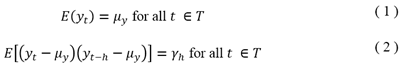Equation (1) simply implies that the expected values of the y process must have a constant mean, so the stationary process must fluctuate around a constant mean defined in µ, no trends are available in the process. Equation
(2) is telling us that variances are time-invariant, so the term γ, doesn’t depend on t but just on the distance h.

In order to get a better notion of stationarity, we define that a stationary process follows the pattern in the next graph. Which was generated using random values over a constant mean of 0, and with a normal probability distribution. The time period sample was n=500 observations.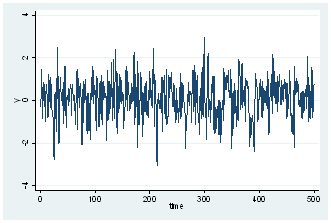The generated process fluctuates around a constant mean, and no tendency is present. How do we confirm if the series is normally distributed? Well, we can perform a histogram over the series. In Stata, the command is histogram y, norm where y is our variable.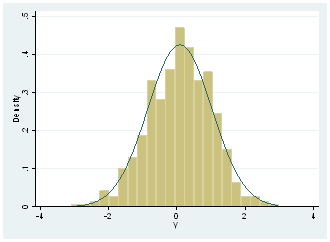The option of ,norm is given in Stata in order to present the actual normal distribution, so we can see that real distribution it’s not far from it. We can graphically affirm that series might present a normal distribution, but in order to confirm it, we need to do a formal test, so we perform Jarque-Bera test with the command sktest y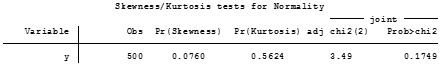The null hypothesis of the test is that normal distribution exists among the y variable And since p-value is bigger than a 5%significance level, we fail to reject null hypothesis and we can say that y variable is normally distributed.

Checking for unit roots also is useful when we’re trying to discover stationarity over a variable, so we perform first, the estimated ideal lag for the test, with varsoc y which will tell us what appropriated lag-length should be used in the ADF test.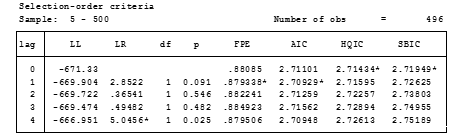Such results, indicate that ADF test over y variable must be done with one lag according to FPE, AIC, while HQIC and SBIC indicate 0 lags. It is the decision of the investigator to select the right information criteria (mostly it is selected when all error criteria are in a specific lag). However, we have a draw of FPE and AIC vs HQIC and SBIC. We will discard FPE since according to Liew (2004) this one is more suitable for samples lower than 120 observations, and thus we will select 0 lag for the test considering our sample size of 500 observations.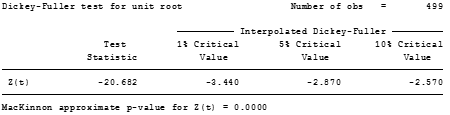Null hypothesis is the existence of unit roots in the variable, so we can strongly reject this and accept that no-unit roots are present. Sometimes this test is used to define stationarity of a respective process, but we need to take in consideration that stationarity involves constant means and normal distributions. We can say for now, that y variable is stationary.

At this point, one could argue Why we need the notion of stationarity over the residuals? This is because stationarity ensures that no spurious regressions are estimated. Now let’s assume we have a model which
follows an I (0) stationary model.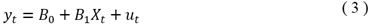And that I (0) variables are y and x, common intuition will tell us that u will be also stationary, but we need to ensure this. Proceeding with our Monte Carlo approaches, we generated the x series with a constant mean which has a normal distribution and that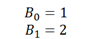with u ~ (0,1) as the Data Generating Process of y expressed in equation (3). Basically u has a mean of 0, and variance of 1. Regressing y on x we got the next result.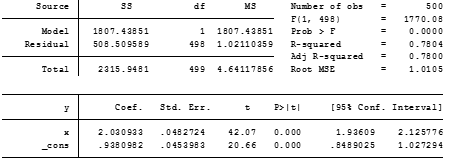We can see that coefficients B_0 and B_1 are approximated 1 and 2 respectively, so it’s almost close to the data generating process and both estimators are statistically significant at 1%. Let’s look at the residuals of the estimated model a little bit closer, we start by predicting the residuals using the command predict u, residuals in order to get the predicted values. Then we perform some of the tests we did before.

Graphic of the residuals with tsline u presentsthe next result, which looks like a stationary process.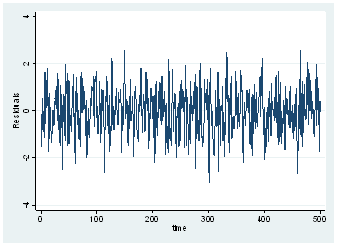A histogram over the residuals, will show the pattern
of normal distribution.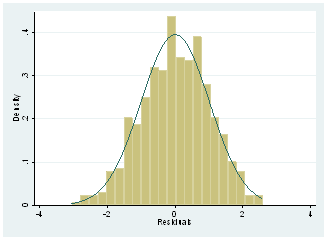And as well, the normality test will confirm this result.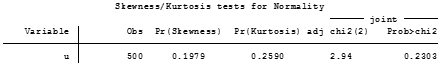Now we need to test that the residuals don’t follow a unit root pattern, a consideration here must be done first before we use ADF test, and is that critical values of the test are not applicable to the residuals. Thus, we cannot fully rely on this test.

In Stata we can recur to the Engle-Granger distribution test of the residuals, to whether accept or reject the idea that residuals are stationary. So, we type egranger y x which provides an accurate estimate of the critical values to evaluate the residuals.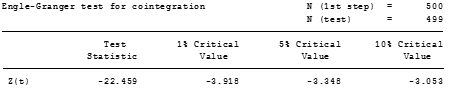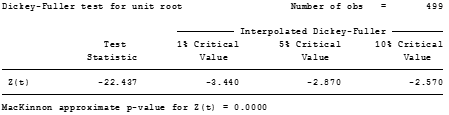As tests evidence, Test statistic is pretty close between ADF test and Engle & Granger test but the critical values are way different. Furthermore, we should rely on the results of the Engle & Granger test. Since Test statistic is bigger than 5% critical value, we can reject the null hypothesis that x and y are not cointegrated, and we can affirm that both variables present over this estimation a long run path of equilibrium. From another view, implies that the residuals are stationary and our regression is not spurious.

This basic idea can be extended with I (1) variables, in order to test whether it exists a long run path and if the regression model in (3) turns to be super consistent. Then long-run approximations with error correction forms can be done for this model where all variables are I (1).

This idea of testing residuals in stationary models is not a formal test used in the literature, however, it can reconfirm that with I (0) models that the regression will not be spurious. And it can also help to contrast long-run relationships.

Note: The package egranger must be installed first ssc install egranger, replace should do the trick. This package parts from the regression model to be estimated, however, it has the failure it cannot be computed with time operators. So, generating first differences or lagged values must be done in separate variables.

Bibliography

Liew, V. (2004). “Which Lag Length Selection Criteria Should We Employ?”. Journal of Economics Bulletin, 1-9. Recuperated from: